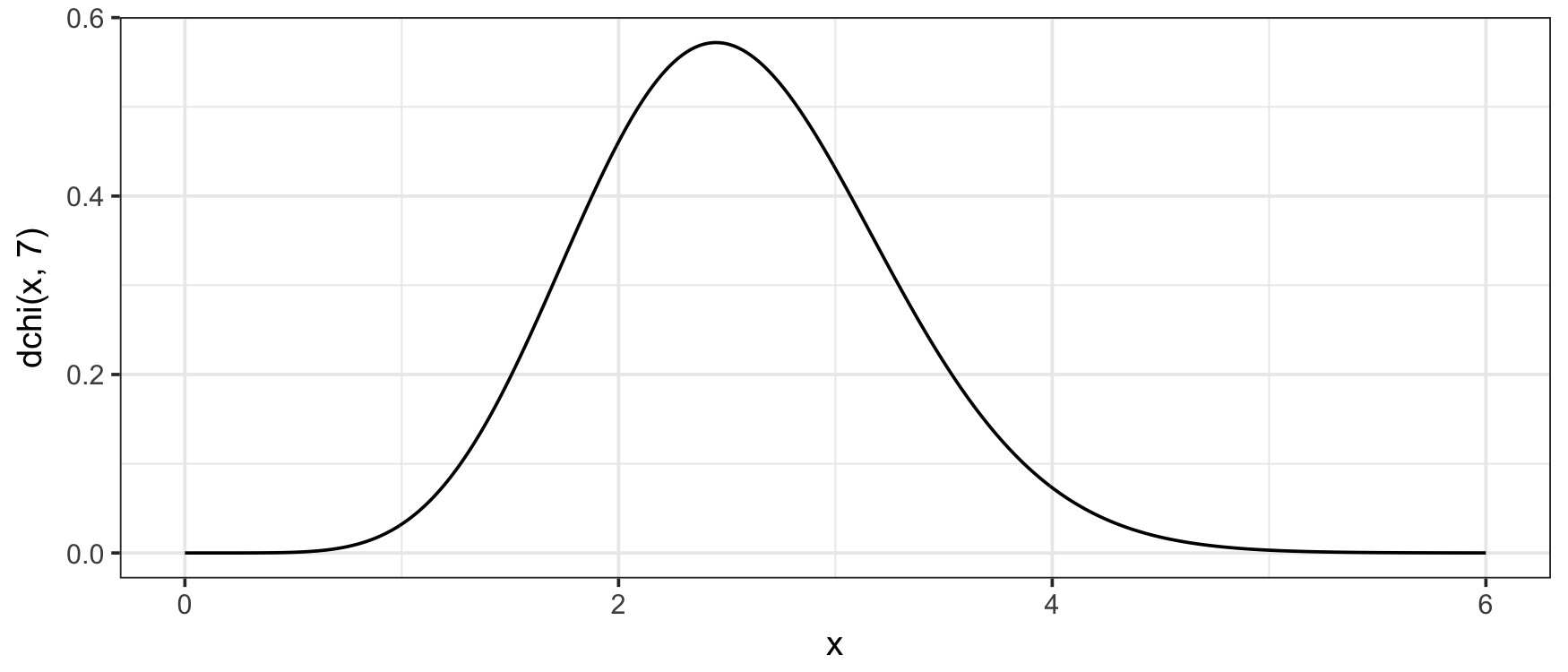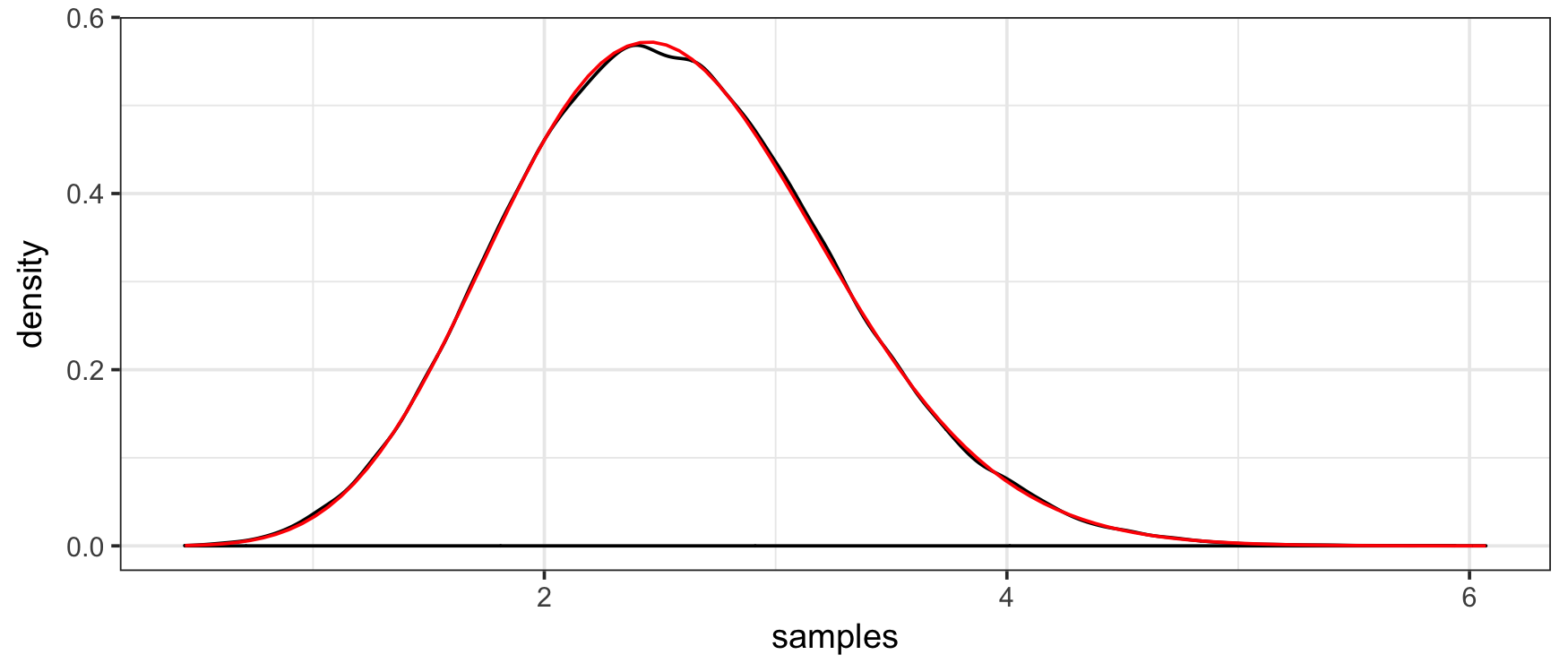# chi

chi implements the `(d/p/q/r)` statistics functions for the chi distribution in R. It is ideal for using in other packages since it is lightweight and leverages the `(d/p/q/r)chisq()` line of functions maintained by CRAN.

### Getting chi

There are two ways to get chi. For the CRAN version, use

``install.packages("chi")``

For the development version, use

``````# install.packages("devtools")
devtools::install_github("dkahle/chi")``````

### The `(d/p/q/r)chi()` functions

The defining property of the chi distribution is that it is the square root of a chi random variable.

The PDF (the f(x)) can be evaluated with the `dchi()` function:

``````library(chi)
library(ggplot2); theme_set(theme_bw())
x <- seq(0, 6, .01)
qplot(x, dchi(x, 7), geom = "line")``````The CDF can be evaluated with the `pchi()` function:

``````f <- function(x) dchi(x, 7)
q <- 2
integrate(f, 0, q)
#  0.2202226 with absolute error < 2.4e-15
(p <- pchi(q, 7))
#   0.2202226``````

The quantile function can be evaluated with `qchi()`:

``````qchi(p, 7) # = q
#   2``````

And random number generation can be performed with `rchi()`:

``````set.seed(1)
rchi(5, 7)
#   2.006520 3.389800 3.349233 2.742686 3.907928``````

`rchi()` can be used to obtain a Monte Carlo estimate of the probability given by `pchi()` above:

``````samples <- rchi(1e5, 7)
mean(samples <= q)
#   0.22172``````

Moreover, we can check the consistency and correctness of the implementation with

``````qplot(samples, geom = "density") +
stat_function(fun = f,  color = "red")``````The Runuran package also implements a sampler from the chi distribution, using the function `Runuran::urchi()`. (It also provides `udchi()`, but not `upchi()` or `uqchi()`.) Here's a comparison of how fast the samplers are, with the punch line being that Runuran is about 3x faster for larger numbers of samples, whereas chi is much faster for smaller samples (e.g. 100 or 1000).

``````library(Runuran)
library(microbenchmark)

# chi::rchi() is much faster for small datasets
microbenchmark(
urchi(1e3, 5),
rchi(1e3, 5)
)
#  Unit: microseconds
#             expr     min       lq     mean   median      uq      max neval
#   urchi(1000, 5) 784.582 808.7745 911.0466 838.4780 929.167 1859.345   100
#    rchi(1000, 5) 109.266 125.1120 143.1345 131.0915 145.558  333.781   100
#   cld
#     b
#    a

# Runuran::urchi is ~3x faster for larger datasets
microbenchmark(
urchi(1e5, 5),
rchi(1e5, 5)
)
#  Unit: milliseconds
#              expr       min        lq      mean    median        uq
#   urchi(1e+05, 5)  4.077995  4.317686  4.765376  4.512885  4.954472
#    rchi(1e+05, 5) 11.912304 12.291699 13.001405 12.669712 13.460673
#         max neval cld
#    6.763741   100  a
#   15.766955   100   b``````